迁移学习让深度学习更容易

+关注继续查看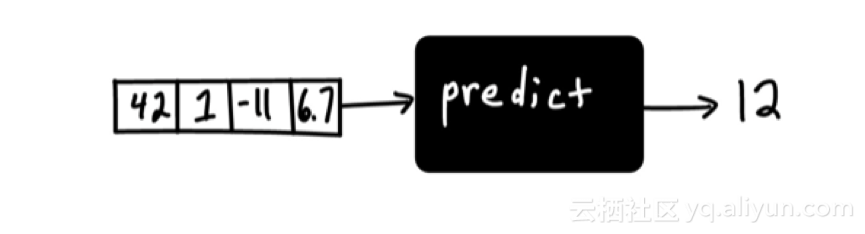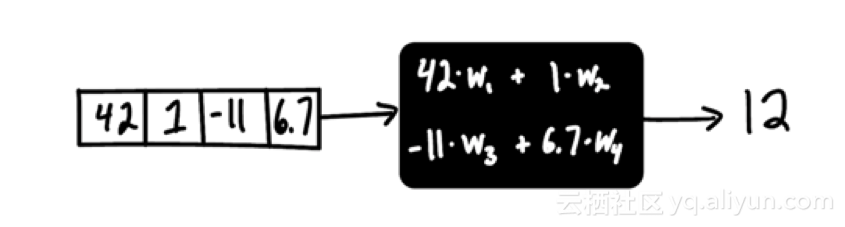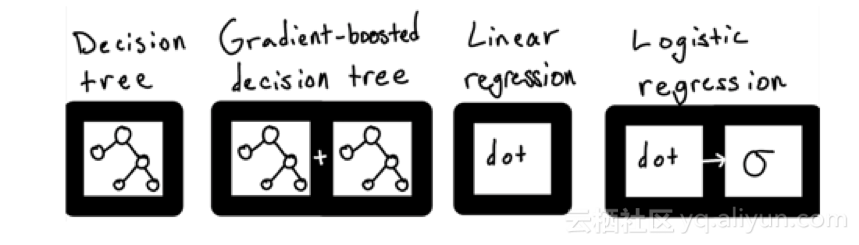clf = RandomForestClassifier()
clf.fit(past_data, labels)
predictions = clf.predict(future_data)

linearModel <- lm(y ~ X, data=pastData)
predictions <- predict(linearModel, futureData)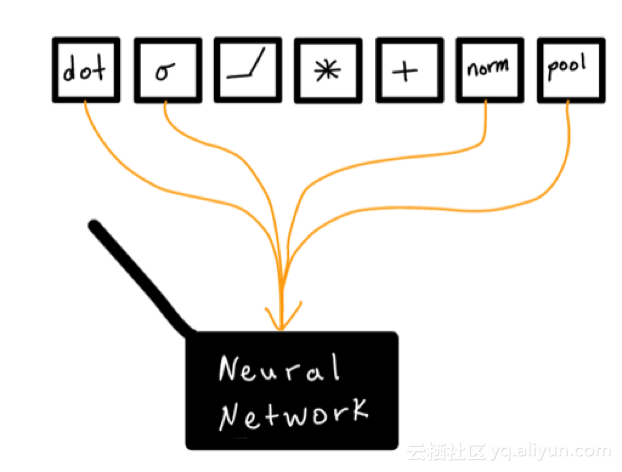class Net(nn.Module):
def __init__(self):
super(Net, self).__init__()
self.conv1 = nn.Conv2d(1, 10, kernel_size=5)
self.conv2 = nn.Conv2d(10, 20, kernel_size=5)
self.conv2_drop = nn.Dropout2d()
self.fc1 = nn.Linear(320, 50)
self.fc2 = nn.Linear(50, 10)

def forward(self, x):
x = F.relu(F.max_pool2d(self.conv1(x), 2))
x = F.relu(F.max_pool2d(self.conv2_drop(self.conv2(x)), 2))
x = x.view(-1, 320)
x = F.relu(self.fc1(x))
x = F.dropout(x, training=self.training)
x = self.fc2(x)
return F.log_softmax(x, dim=1)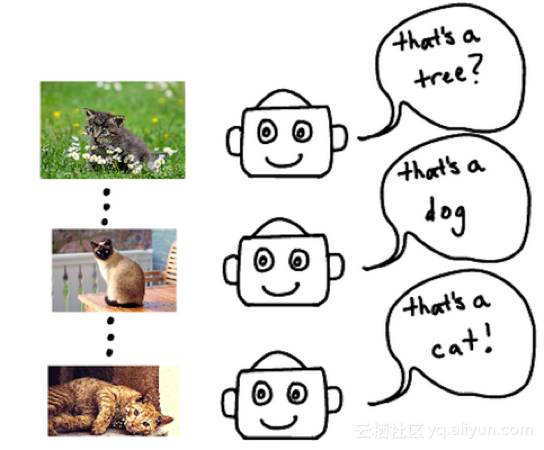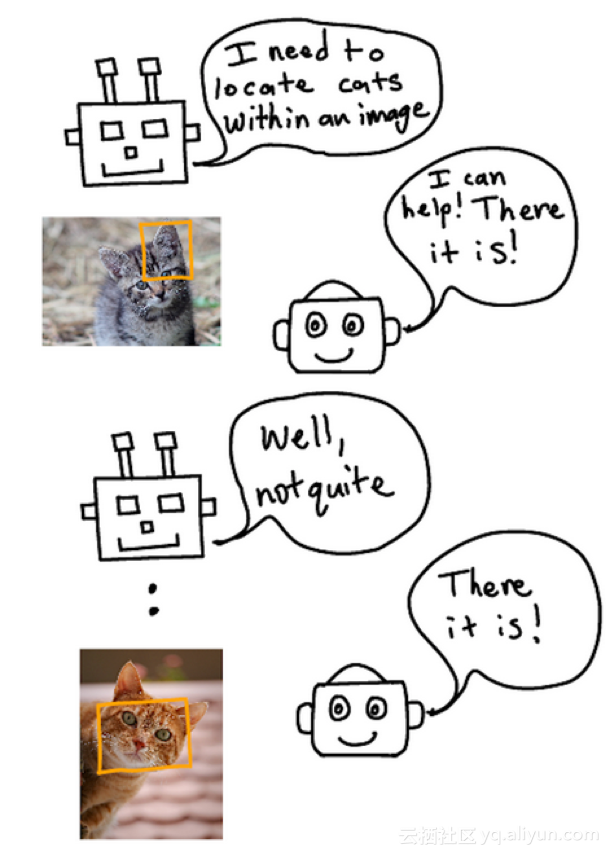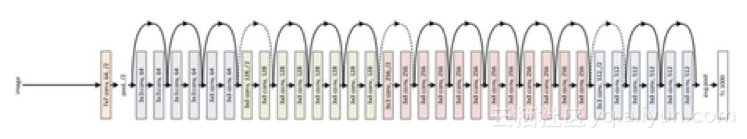1102 0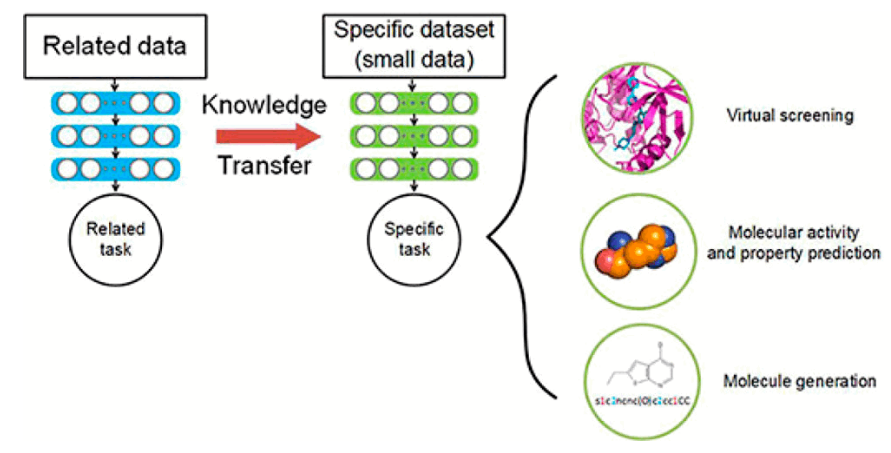JMC | 药物发现中的迁移学习
JMC | 药物发现中的迁移学习
11 0Additional Resources Official Resources: TensorFlow Python API TensorFlow on Github TensorFlow Tutorials Udacity Deep Learning Class TensorFlow Playg...
908 01015 0977 011894 02645 0TiDB 源代码学习: ranger 模块代码分析

20 01153 0
【方向】

707

5

《2021云上架构与运维峰会演讲合集》

《零基础CSS入门教程》

《零基础HTML入门教程》